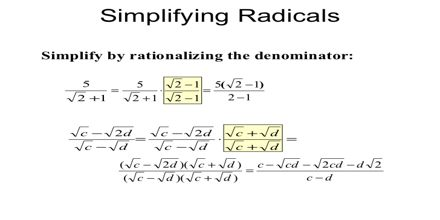Mathematic

# Rationalizing the DenominatorA fraction that contains a radical in its denominator can be written as an equivalent fraction with a rational denominator. This lecture focus to present on Rationalizing the Denominator. “Rationalizing the denominator” is when we move a root (like a square root or cube root) from the bottom of a fraction to the top. There is an agreement in mathematics that we don’t leave a radical in the denominator of a fraction.